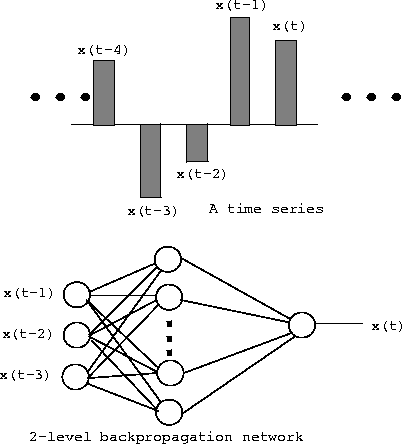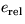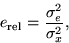INSTRUCTIONS FOR THE PROJECT OF NEURAL COMPUTING
TIME SERIES PREDICTION

# General Information

The project in neural computing is done by using the MATLAB program [2,3,4] with some extension functions for neural computing. The extension functions used for neural computing are a software package called Neural Network Toolbox . The project can be done by using any computer with MATLAB and Neural Network Toolbox (which is a set of MATLAB-functions). MATLAB and Neural Network Toolbox are installed at workstations in Maarintalo. The manuals of the toolbox can also be found in Maarintalo administration.

# Files

The file containing the time series can be found here or at Maarintalo workstations in directory /p/edu/tik-61.170/neural/timeser. Name of the file containing the time series in MATLAB-format (binary) is ts.mat (the name of the vector in MATLAB is ts).

# Exercises

## Structure of the exercises

In the project, the time series is predicted by using some preceding values to predict the next value of the time series (for example 3 preceding values). A part of the time series is selected to be the training set (in maximum half of the time series) and the other part is used for testing the network. A 2- and 3-layer multi-layer perceptron (MLP)  network are then trained to predict the next value of the time series by using the preceding values as input. The training algorithm used here is backpropagation . Try at least with four different networks with different amount of layers (2 and 3) and with different amounts of hidden layer neurons. Do these changes have effect on the learning rate and the final error? Compare also different training functions (at least 2). Are there any differences?## Reporting

The report begins with a cover page including course code, course name, name of the exercise (time series prediction), your name, your department, your email address, student number and return date. In the report, the chosen networks and their parameters are described and reasoned. The predicting results are presented for the training set and for the test set. One way to present the results is to plot the original time series and the predicted time series in the same figure (different figures for the training set and the test set). A shorter period of time series is recommended to be printed (for example 100 points) to keep the figures clear. Calculate also the relative error for the training set, the test set and for the whole time series. The relative erroris defined using error and correct signal'' variances:where e is error and x is the correct signal. The MATLAB-codes used for the project should be appended to the report. The MATLAB-codes should be clearly commented and readable.

## References

1
S. Haykin. Neural Networks - a Comprehensive Foundation, Prentice-Hall 1998

2
S.K. Kivelä. MATLAB-opas, 2nd edition 1988

3
A Prewiev of MATLAB, The Math Works Inc. 1989

4
C. Moler et al. PC-MATLAB User's Guide, The Math Works Inc. 1987

5
Neural Network Toolbox User's Manual, The Math Works Inc. 1992